Start your cart here complete your order on Maillot-Erable or Japon-Import and reduce your shipping costs##### The Japanese Bonsai specialist
Direct order Contact Help / Services Newsletter Payez en 3X sans frais dès 135€ d'achat# Tool kit n°2

› Bonsai tools › Bonsai toolkits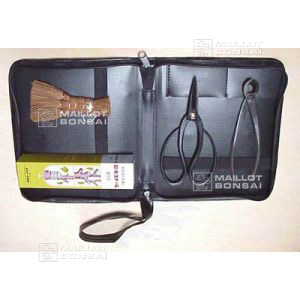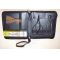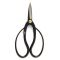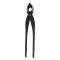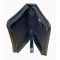ref. : 5454

90,00

Available quantity : 3Order

###### Description

1 kit in black skai 235*205 mm. This is a brilliant starter kit for bonsaists and a good gift idea. The smart faux leather zipped case comes with top quality tools made in Japan, including a coconut palm brush, a tube of healing putty, 1 pair of large handled scissors and 1 concave tool 180 mm. The case can accommodate 7 tools in its elasticated compartments and the wrist strap makes it easy to carry. Normal price 99.35 euros, promo price: 90 euros.

#tool 3.4 #toolkits 2.8 #bonsai 2.6 #price 2.5 #euros 2.5 #tools 2.5 #case 2.4 #compartments 2.2 #accommodate 2.1 #elasticated 2.1

Formule
(( ROUND((CHAR_LENGTH(b.article_nom)-CHAR_LENGTH(REPLACE(b.article_nom, 'tool', '')))/LENGTH('tool')) + ROUND((CHAR_LENGTH(b.article_description)-CHAR_LENGTH(REPLACE(b.article_description, 'tool', '')))/LENGTH('tool')) ) * 3.4) + (( ROUND((CHAR_LENGTH(b.article_nom)-CHAR_LENGTH(REPLACE(b.article_nom, 'price', '')))/LENGTH('price')) + ROUND((CHAR_LENGTH(b.article_description)-CHAR_LENGTH(REPLACE(b.article_description, 'price', '')))/LENGTH('price')) ) * 2.5) + (( ROUND((CHAR_LENGTH(b.article_nom)-CHAR_LENGTH(REPLACE(b.article_nom, 'tools', '')))/LENGTH('tools')) + ROUND((CHAR_LENGTH(b.article_description)-CHAR_LENGTH(REPLACE(b.article_description, 'tools', '')))/LENGTH('tools')) ) * 2.5) + (( ROUND((CHAR_LENGTH(b.article_nom)-CHAR_LENGTH(REPLACE(b.article_nom, 'euros', '')))/LENGTH('euros')) + ROUND((CHAR_LENGTH(b.article_description)-CHAR_LENGTH(REPLACE(b.article_description, 'euros', '')))/LENGTH('euros')) ) * 2.5) + (( ROUND((CHAR_LENGTH(b.article_nom)-CHAR_LENGTH(REPLACE(b.article_nom, 'case', '')))/LENGTH('case')) + ROUND((CHAR_LENGTH(b.article_description)-CHAR_LENGTH(REPLACE(b.article_description, 'case', '')))/LENGTH('case')) ) * 2.4) + (( ROUND((CHAR_LENGTH(b.article_nom)-CHAR_LENGTH(REPLACE(b.article_nom, 'compartments', '')))/LENGTH('compartments')) + ROUND((CHAR_LENGTH(b.article_description)-CHAR_LENGTH(REPLACE(b.article_description, 'compartments', '')))/LENGTH('compartments')) ) * 2.2) + (( ROUND((CHAR_LENGTH(b.article_nom)-CHAR_LENGTH(REPLACE(b.article_nom, 'accommodate', '')))/LENGTH('accommodate')) + ROUND((CHAR_LENGTH(b.article_description)-CHAR_LENGTH(REPLACE(b.article_description, 'accommodate', '')))/LENGTH('accommodate')) ) * 2.1) + (( ROUND((CHAR_LENGTH(b.article_nom)-CHAR_LENGTH(REPLACE(b.article_nom, 'elasticated', '')))/LENGTH('elasticated')) + ROUND((CHAR_LENGTH(b.article_description)-CHAR_LENGTH(REPLACE(b.article_description, 'elasticated', '')))/LENGTH('elasticated')) ) * 2.1) + (( ROUND((CHAR_LENGTH(b.article_nom)-CHAR_LENGTH(REPLACE(b.article_nom, 'bonsaists', '')))/LENGTH('bonsaists')) + ROUND((CHAR_LENGTH(b.article_description)-CHAR_LENGTH(REPLACE(b.article_description, 'bonsaists', '')))/LENGTH('bonsaists')) ) * 1.9) + (( ROUND((CHAR_LENGTH(b.article_nom)-CHAR_LENGTH(REPLACE(b.article_nom, 'brilliant', '')))/LENGTH('brilliant')) + ROUND((CHAR_LENGTH(b.article_description)-CHAR_LENGTH(REPLACE(b.article_description, 'brilliant', '')))/LENGTH('brilliant')) ) * 1.9)

## Secure payment## Delivery

Our logistic partners :